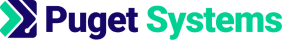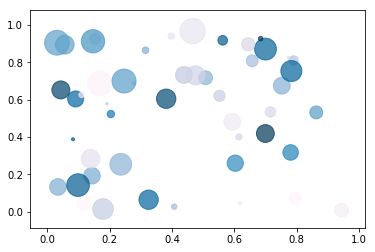https://www.pugetsystems.com

# Machine Learning and Data Science: Introduction

Written on April 28, 2017 by Dr Donald Kinghorn
Share:

This is the first post in a series that I have wanted to do for some time. Machine Learning and Data Science, fascinating! This is one of the most interesting areas of endeavor today. It has truly blossomed over the last few years. There are numerous high quality tools and frameworks for handling and working with data sets, a seemingly endless number of application domains, and lots and lots of data. Data Science has become "a thing". Many Universities are offering graduate programs in "Data Science". It has existed for ages as part of Statistics, Informatics, Business Intelligence/Analytics, Applied Mathematics, etc., but it is now taking on a multidisciplinary life of its own.

In this series I'll be exploring the algorithms and tools of Machine Learning and Data Science. It will be tutorials, guides, how-to, reviews, "real world" application, and whatever I feel like writing about. It will be documenting my own journey. Even though I am familiar with the mathematics many of the algorithms and tools are new to me. This is partly a revival of the fascination I had with neural networks as a graduate student in the mid 1990's but never had the time to pursue. It's about time I did it!

## What is Machine Learning¶

I wrote a blog post in 2016 titled What is Machine Learning. I looked at it again and decided I like the definition I came up with so here it is.

Machine Learning -- Machine Learning (ML) is a multidisciplinary field focused on implementing computer algorithms capable of drawing predictive insight from static or dynamic data sources using analytic or probabilistic models and using refinement via training and feedback. It make use of pattern recognition, artificial intelligence learning methods, and statistical data modeling. Learning is achieved in two primary ways; Supervised learning, where the desired outcome is known and an annotated data set or measured values are used to train/fit a model to accurately predict values or labels outside of the training set. This is basically “regression” for values and “classification” for labels. Unsupervised learning uses “unlabeled” data and seeks to find inherent partitions or clusters of characteristics present in the data. ML draws from the fields of computer science, statistics, probability, applied mathematics, optimization, information theory, graph and network theory, biology, and neuroscience.

## What is Data Science¶

In my view Machine Learning and is the primary "What", Data Science is the primary "How". Data Science has historically been just another name for Statistics. However, I feel that it has become much more than that! After about 2010 everything has changed. It used to be that the largest data set that a Statistician might have to work with was the US census data. That's a few 100 gigabytes, not small, but not so much by todays measure of "big data". The reality of any "real world" Machine Learning project is that a large part of the time and effort is going to put into getting usable data ready for some machine learning algorithm. There is also the problem of just trying to understand what useful information your data has to offer and how you are going to model that. Data visualization is also an important part of that.

Here's my take on a definition for "Data Science";

Data Science -- Data Science is an emerging field of study establishing formalism and "best practices" for manipulating and extracting useful information from, possibly massive, data sets. It is a widely multidisciplinary field incorporating elements of;

• Statistics and Probability
• Machine Learning and Artificial Intelligence
• Data Visualization
• Numerical Analysis, Optimization, Applied Mathematics
• Computer Science, DevOps, Programming, HPC
• Domain Specific Knowledge (Sciences, Business, Finance, etc.)

It is defining best practices for, Data Analysis and Reproducible Research.

### Reproducible Research¶

This is important! There is a crisis in research reproducibility. The further away research literature is from peer reviewed pure mathematics the more likely it is to irreproducible or just plain junk. The Data Science community seems to be making a serious effort to use and establish reproducible methodology.

• Literate Programming (Documentation and code together)
• Source control and Continuous Integration (DevOps!)
• Environment reproducibility via Containerization with Docker ;-) (the wink is for those of you that have read my Docker posts)
• Well maintained open source tools

The Data Science community is remarkably "open" considering that a lot of Data Science gets done by large commercial interest's.

### Jupyter Notebooks¶

In keeping with the spirit of reproducibility I will be writing this series of blog posts as Jupyter notebooks and making them available on GitHub. This blog post so far is markdown code in a notebook cell.

In Jupyter I can easily add LaTeX code like this little formula

$$R = \sum_{t=0}^{q-1}\frac{1}{q}\gamma_{3}\left( p-q \right) \gamma_{3} \left( t \right) \left( 1-\frac{c}{ab}\right) ^{q-t}$$

and have it display nicely in the notebook,

$$R = \sum_{t=0}^{q-1}\frac{1}{q}\gamma_{3}\left( p-q \right) \gamma_{3} \left( t \right) \left( 1-\frac{c}{ab}\right) ^{q-t}$$

I can write Python and execute it in the document

In :
print("Hello from Python3")

Hello from Python3


Make a plot

In :
%matplotlib inline
import numpy as np
import matplotlib.pyplot as plt

N = 50
x = np.random.rand(N)
y = np.random.rand(N)
colors = np.random.rand(N)
area = np.pi * (15 * np.random.rand(N))**2
plt.scatter(x, y, s=area, c=colors, alpha=0.7, cmap='PuBu')
plt.show()Good stuff like that ... and then use the notebook to generate HTML for the blog post.

Happy computing! --dbk

Tags: Machine Learning, Data Science, Python, Jupyter notebook, Programming